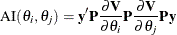# The HPMIXED Procedure

### Computing and Maximizing the Likelihood

In computing the restricted likelihood function given previously, the determinants of the matrices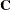andcan be obtained effectively by using Cholesky decomposition. The quadratic term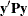can be expressed in terms of solutions of mixed model equations as follows: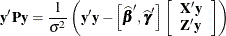By default, the HPMIXED procedure profiles out the residual variance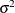from the parameter vector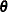. Let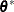be the new parameter vector such that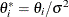. The profiled objective function becomes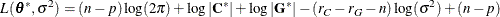where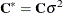and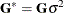are the profiled versions ofand,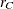and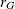are the ranks ofand. Minimizing analytically foryields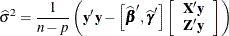Optimizing the likelihood calls for derivatives with respect to the parameters. The first and second derivatives of the log-likelihood function L with respect to scalar variance components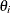and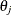are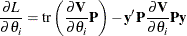and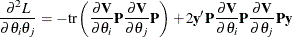The default quasi-Newton method of optimization for the HPMIXED procedure requires only first derivatives of the log likelihood, and these are readily derived by solving the mixed model equations. For example, when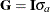, the first derivative of the log likelihood with respect to the parameter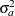can be computed as follows: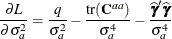where q is the size of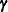vector and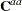is the part of the g-inverse of the mixed model equation coefficient matrixcorresponding to the random effect.

The second derivative of the log likelihood needs to be computed only if you specify certain nondefault optimization techniques in the NLOPTIONS statement, namely TECH=NEWRAP, TECH=NRRIDG, or TECH=TRUREG; see NLOPTIONS Statement in Chapter 19: Shared Concepts and Topics, for more information about optimization techniques. For these second-derivative-based optimization techniques, the HPMIXED procedure does not actually use the true second derivative matrix, or observed information matrix, as defined earlier. Instead, it uses an alternative matrix that is more efficient to compute for large problems and that can be more stable. This alternative is called the average information matrix, and it is defined as follows. The expected value of the second derivative is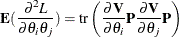It is this trace that is computationally inefficient to evaluate. But if you average the expected information matrix defined by this formula with the observed information matrix defined by the preceding formula for the true second derivative, then the trace term cancels, leaving just a quadratic expression in. This quadratic expression defines the average information (Johnson and Thompson 1995) with respect toand: